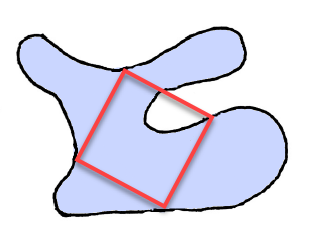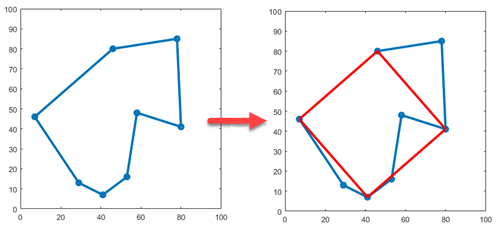# Problem 46565. Find an inscribed square on a closed curve

Here's an unproven conjecture from geometry: Every simple closed curve in a plane contains all four vertices of some square.It's kind of remarkable. As far as we can tell right now, you can put at least one square on every single closed curve. Inspired by this, I made a simple discrete Cody-fied version of the problem. (And by the way, I'm not claiming it's mathematically equivalent...)
Given a list of points in [x y] format, return four indices into that list that form a square.
xy = [ 29 13
41 7
53 16
58 48
80 41
78 85
46 80
7 46 ]
Then the answer would be the indices given in ix. These indices point to the rows that contain the four vertices of the square.
ix = [ 2 5 7 8 ]You can return the indices in any order that honors the cycle around the square, and the answer is not necessarily unique. The test suite just verifies that what you return is indeed a square.

### Solution Stats

48.65% Correct | 51.35% Incorrect
Last Solution submitted on Oct 14, 2023

### Community Treasure Hunt

Find the treasures in MATLAB Central and discover how the community can help you!

Start Hunting!